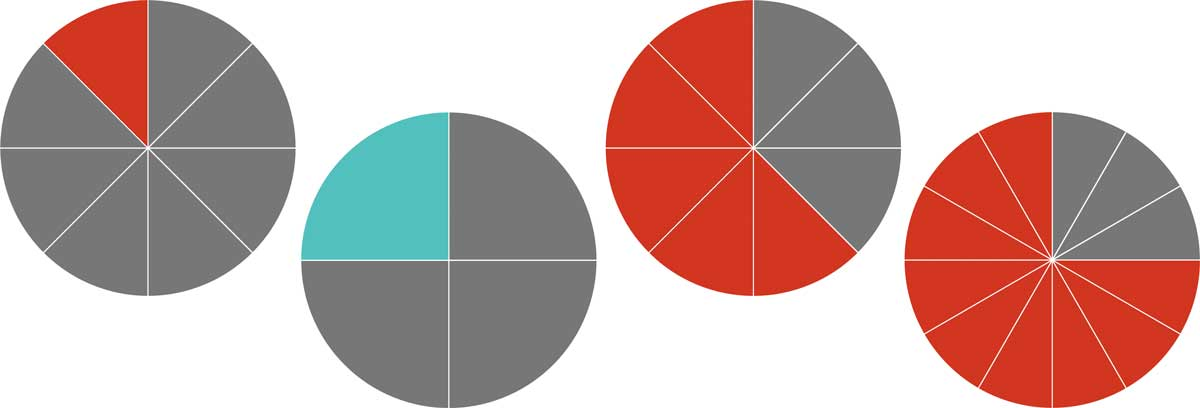# Fraction Calculators and ToolsUse our fraction calculators and tools to help add, subtract, multiply, and divide fractions, compare fractions, solve fractions in algebraic equations reduce fractions, convert fractions to decimals and percentages, or convert decimals to fractions.